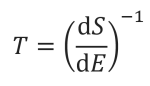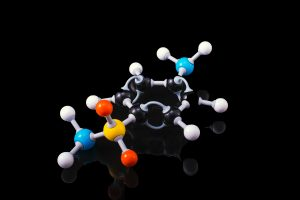# Hotter than Hot: Negative Temperatures

Fields covered: Physics, Thermodynamics, Energy

Related Articles:
§Below Absolute Zero: Negative Temperatures Explained

The normal temperature scale we see on a daily basis stretches from the refrigerator to the stove. The more scientifically inclined might be aware that there is a lower limit to the temperatures we can achieve: Absolute zero. Where did this come from?

Temperature is typically defined, in classical thermodynamics: as the amount of transferable kinetic energy (motional energy) the atoms of an object have. This automatically sets a definition for the lower limit for temperature – absolute zero – the point at which the atoms in an object are not moving. This is a fundamental principle and thus there exists a temperature unit that is defined around this limit. The Kelvin (K) is set such that it begins at absolute zero.

Given this definition of the Kelvin, how is it possible that we achieve negative temperatures then? It’s not as if we can imbue an object with negative energy, or have them vibrate with negative motion. It seems like a ridiculous idea.

*

The trick lies in the more rigorous definition of temperature, embedded in statistical mechanics. Let’s first begin with entropy. It is a measure of the amount of “disorder” in a given system. Temperature can then be defined as a function of change in ‘disorder’ of a system given some change in energy:Where T is the temperature in Kelvin, dS represents the change in entropy (disorder) and, dE is the change in energy.

Consider a normal system, such as a pot of water. When you add energy to it, such as by heating it up, you increase the amount of disorder. Think water boiling and steam forming. This increase in entropy is related to the increase in temperature of the system. A system that exhibits an increase in entropy with an increase in energy has a positive temperature.

We can start to get an inkling of how negative temperatures may arise. A system whose disorder decreases with increasing energy exhibits negative temperatures. But how can such a system arise?

Consider another system, made of atoms that can only exist at certain energy levels, and no other (See gif). At first, we can see that as energy increases, the disorder in the system increases. Entropy reaches the maximum when the atoms are scattered randomly. This is the point of infinite temperature. However, as there is a maximum energy level that the atoms can exist at, order reasserts itself as energy increases further.

There are certain systems in which this phenomenon can arise, such as in lasers, where there are limited energy levels. In our day-to-day world, we don’t see this happening. The kinetic energy of particles we encounter is not limited to a maximum value. However, we can appreciate how quirks of the world around us can lead to deviations from what we think are facts.

## Other Briefs## Hotter than Hot: Negative Temperatures## Dumbbell-Shaped Machine Decodes Polystyrene## Lead Halide Perovskites: Rainbow in a Bottle## A Bet You Might Not Want To Take – Is A Coin Toss Ever Fair?

Do you not think that there are things which you cannot understand, and yet which are; that some people

see things that others cannot?”

Special Programme in Science
Average rating:
0 reviews